Most Affordable JEE | NEET | 8,9,10 Preparation by Kota's Top IITian Doctor Faculties

# Sequence-Series - JEE Main Previous Year Question with SolutionsJEE Main Previous Year Question of Math with Solutions are available at eSaral. Practicing JEE Main Previous Year Papers Questions of mathematics will help the JEE aspirants in realizing the question pattern as well as help in analyzing weak & strong areas. eSaral helps the students in clearing and understanding each topic in a better way. eSaral is providing complete chapter-wise notes of Class 11th and 12th both for all subjects. Besides this, eSaral also offers NCERT Solutions, Previous year questions for JEE Main and Advance, Practice questions, Test Series for JEE Main, JEE Advanced and NEET, Important questions of Physics, Chemistry, Math, and Biology and many more. Download eSaral app for free study material and video tutorials.
Q. The sum to infinity of the series $1+\frac{2}{3}+\frac{6}{3^{2}}+\frac{10}{3^{3}}+\frac{14}{3^{4}}+\ldots .$ is :- (1) 4          (2) 6          (3) 2           (4) 3 [AIEEE-2009]
Ans. (4)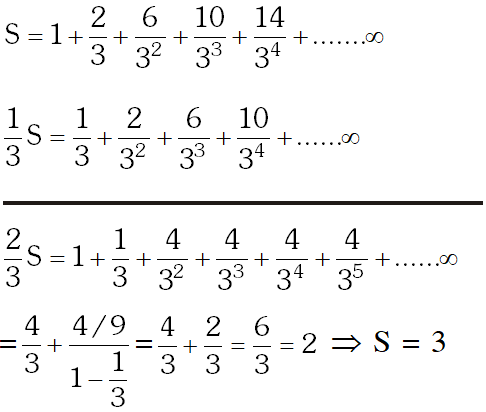Q. A person is to count 4500 currency notes. Let a denote the number of notes he counts in the $\mathrm{n}^{\mathrm{th}}$ minute. If $\mathrm{a}_{1}=\mathrm{a}_{2}=\ldots=\mathrm{a}_{10}=150$ and $\mathrm{a}_{10} \mathrm{a}_{11}, \ldots$ are in an AP with common difference - $2,$ then the time taken by him to count all notes is :- (1) 24 minutes        (2) 34 minutes          (3) 125 minutes         (4) 135 minutes [AIEEE-2010]
Ans. (2)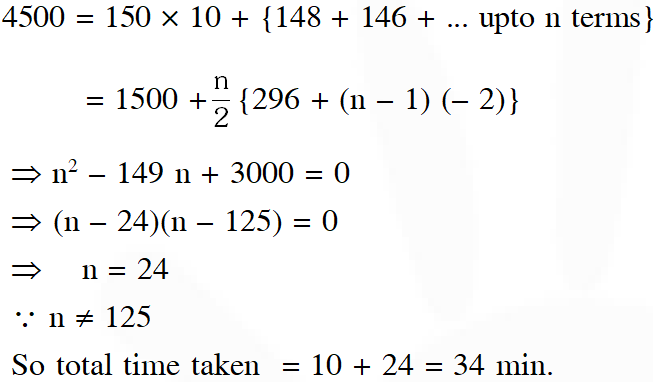Q. A man saves Rs. 200 in each of the first three months of his service. In each of the subsequent months his saving increases by Rs. 40 more the saving of immediately previous month. His total saving from the start of service will be Rs. 11040 after :- (1) 20 months        (2) 21 months            (3) 18 months        (4) 19 months [AIEEE-2011]
Ans. (2) Saving after first 3 month $=600$ $600+\left\{\frac{240+280+\ldots .}{\text { let } n \text { month }}\right\}=11040$ $| 240+280+\ldots . .$ n terms $|=10440$ n/2 $[480+\ldots . . . \text { n terms }]=10440$ n/2 $[480+(n-1) 40]=10440$ n $\{440+40 n\}=20880$ $\mathrm{n}^{2}+11 \mathrm{n}-522=0$ $\mathrm{n}=18,-29 \quad(-29 \text { rejected })$ Total months $=\mathrm{n}+3$ 18 $+3=21$ Months
Q. Let $a_{n}$ be the $n^{\text {th }}$ term of an A.P. If $\sum_{r=1}^{100} a_{2 r}=\alpha$ and $\sum_{r=1}^{100} a_{2 r-1}=\beta,$ then the common difference of the A.P. is : (1) $\frac{\alpha-\beta}{200}$ (2) $\alpha-\beta$ (3) $\frac{\alpha-\beta}{100}$ (4) $\beta-\alpha$ [AIEEE-2011]
Ans. (3)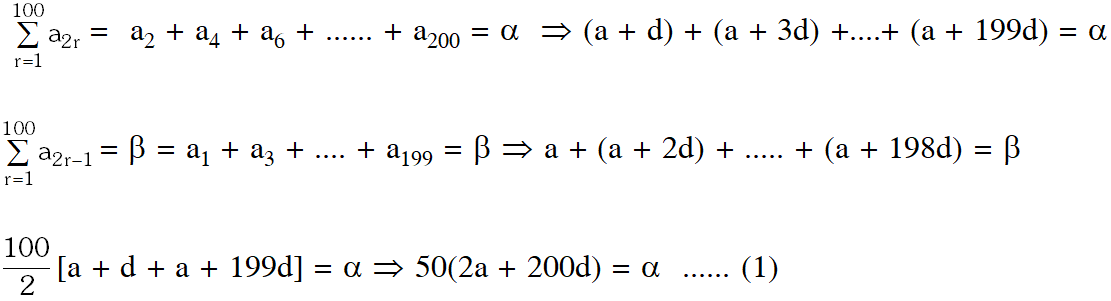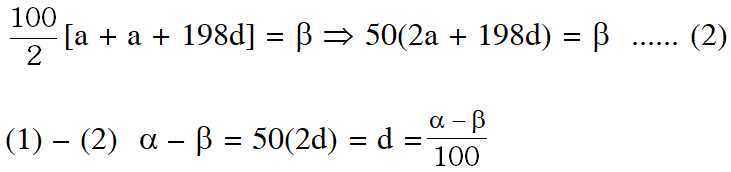Q. Statement-1: The sum of the series $1+(1+2+4)+(4+6+9)+(9+12+16)$ $\quad+\ldots \ldots+(361+380+400)$ is $8000 .$ Statement-2: $\sum_{\mathrm{k}=1}^{\mathrm{n}}\left(\mathrm{k}^{3}-(\mathrm{k}-1)^{3}\right)=\mathrm{n}^{3},$ for any natural number $\mathrm{n}$ (1) Statement-1 is true, Statement-2 is false. (2) Statement- 1 is false, Statement- 2 is true. (3) Statement- 1 is true, Statement-2 is true ; Statement-2 is a correct explanation for Statement-1. (4) Statement-1 is true, Statement-2 is true; Statement-2 is not a correct explanation for Statement- - $1 .$ [AIEEE-2012]
Ans. (4) Statement-1: $\left(1^{3}-0^{3}\right)+\left(2^{3}-1^{3}\right)+\left(3^{3}-2^{3}\right)+\ldots+\left(20^{3}-19^{3}\right)=20^{3}=8000$ Statement-l is true. Statement-2: $\sum_{k=1}^{n} k^{3}-(k-1)^{3}=\left(1^{3}-0^{3}\right)+\left(2^{3}-1^{3}\right)+\left(3^{3}-2^{3}\right)+\ldots . n^{3}+(n-1)^{3}=n^{3}$ Statement-2 is true and Statement-2 is a correct explanation of Statement- $1 .$
Q. If 100 times the 100th term of an A.P. with non-zero common difference equals the 50 times its 50th term, then the 150th term of this A.P. is : (1) zero             (2) –150           (3) 150 times its               50th term (4) 150 [AIEEE-2012]
Ans. (1)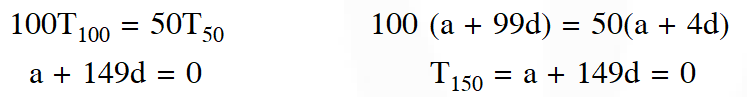Q. The sum of first 20 terms of the sequence 0.7, 0.77, 0.777, ......, is : (1) $\frac{7}{81}\left(179-10^{-20}\right)$ (2) $\frac{7}{9}\left(99-10^{-20}\right)$ (3) $\frac{7}{81}\left(179+10^{-20}\right)$ (4) $\frac{7}{9}\left(99-10^{-20}\right)$ [JEE(Main)-2013]
Ans. (3) $S=\frac{7}{10}+\frac{77}{100}+\frac{777}{1000}+\ldots \ldots \quad S=\frac{7}{9}\left\{\frac{10-1}{10}+\frac{100-1}{100}+\frac{1000-1}{1000}+\ldots\right\}$ $=\frac{7}{9}\left\{20-\frac{1}{10}\left(\frac{1-10^{-20}}{9 / 10}\right)\right\}=\frac{7}{9}\left\{20-\frac{1}{9}\left(1-10^{-20}\right)\right\}=\frac{7}{81}\left(179+10^{-20}\right)$
Q. Let $\alpha$ and $\beta$ be the roots of equation $\mathrm{px}^{2}+\mathrm{qx}+\mathrm{r}=0, \mathrm{p} \neq 0 .$ If $\mathrm{p}, \mathrm{q}, \mathrm{r}$ are in A.P. and $\frac{1}{\alpha}+\frac{1}{\beta}=4,$ then the value of $|\alpha-\beta|$ is: (1) $\frac{\sqrt{61}}{9}$ (2) $\frac{2 \sqrt{17}}{9}$ (3) $\frac{\sqrt{34}}{9}$ (4) $\frac{2 \sqrt{13}}{9}$ [JEE(Main)-2014]
Ans. (4) $\frac{\alpha+\beta}{\alpha \beta}=4=-\frac{q}{r} \Rightarrow q=-4 r$ $\because p, q \& r$ are in A.P $2 q=p+r$ $\Rightarrow \quad-8 r=p+r \Rightarrow p=-9 r$ $|\alpha-\beta|=\sqrt{(\alpha+\beta)^{2}-4 \alpha \beta}=\sqrt{\frac{16 r^{2}+36 r^{2}}{p^{2}}}=\sqrt{\frac{52 r^{2}}{p^{2}}}=\sqrt{\frac{52}{81}}=\frac{2 \sqrt{13}}{9}$
Q. Three positive numbers form an increasing G.P. If the middle term in this G.P. is doubled, the new numbers are in A.P. Then the common ratio of the G.P. is : (1) $\sqrt{2}+\sqrt{3}$ (2) $3+\sqrt{2}$ (3) $2-\sqrt{3}$ (4) $2+\sqrt{3}$ [JEE(Main)-2014]
Ans. (4) Let a, ar, ar $^{2}$ are in G.P $\therefore \quad$ a, 2 ar, $\operatorname{ar}^{2}$ are in AP $\Rightarrow \quad 4$ ar $=a+a r^{2}$ $\Rightarrow \quad r^{2}-4 r+1=0$ $\Rightarrow r=2+\sqrt{3}, 2-\sqrt{3}$ since GP is an increasing G.P $\Rightarrow r=2+\sqrt{3}$
Q. If $(10)^{9}+2(11)^{1}(10)^{8}+3(11)^{2}(10)^{7}+\ldots \ldots+10(11)^{9}=\mathrm{k}(10)^{9},$ then $\mathrm{k}$ is equal to : (1) $\frac{121}{10}$ (2) $\frac{441}{100}$ (3) 100 (4) 110 [JEE(Main)-2014]
Ans. (3) $\mathrm{S}=10^{9}+2(11)^{1}(10)^{8}+3(11)^{2}(10)^{7}+\ldots+10(11)^{9}$ $\frac{11}{10} \mathrm{S}=(11)(10)^{8}+2(11)^{2}(10)^{7}+\ldots 11^{10}$ $-\frac{\mathrm{S}}{10}=10^{9}+\left(11.10^{8}+11^{2} .10^{7}+\ldots .+11^{9}\right)-11^{10}$ \begin{aligned}-\frac{\mathrm{S}}{10} &=10^{9}+11.108^{8} \frac{\left(1-\left(\frac{11}{10}\right)^{9}\right)}{\left(1-\frac{11}{10}\right)}-11^{10} \\ &=10^{9}+10^{8} \cdot 11 \frac{\left(10^{9}-11^{9}\right)}{10^{9}(-1)} \cdot 10-11^{10} \\ &=10^{9}+11\left(11^{9}-11^{9}\right) \cdot 10-11^{10} \\ &=10^{9}+11\left(11^{9}-10^{9}\right)-11^{10} \\ \mathrm{S} &=10^{11}=\mathrm{K} 10^{9} \\ \Rightarrow \mathrm{K} &=100 \end{aligned}
Q. If $\mathrm{m}$ is the A.M. of two distinct real numbers 1 and $\mathrm{n}(1, \mathrm{n}>1)$ and $\mathrm{G}_{1}, \mathrm{G}_{2}$ and $\mathrm{G}_{3}$ are three geometric means between 1 and $\mathrm{n}$, then $\mathrm{G}_{1}^{4}+2 \mathrm{G}_{2}^{4}+\mathrm{G}_{3}^{4}$ equals – (1) $4 \operatorname{lmn}^{2}$ (2) $41^{2} \mathrm{m}^{2} \mathrm{n}^{2}$ (3) $4 \mathrm{l}^{2} \mathrm{mn}$ (4) $4 \ln ^{2} n$ [JEE(Main)-2015]
Ans. (4) $\ell, \mathrm{G}_{1}, \mathrm{G}_{2}, \mathrm{G}_{3}, \mathrm{n}$ in G.P. Let $\mathrm{r}$ be the common ratio $\Rightarrow \mathrm{r}^{4}=\frac{\mathrm{n}}{\ell}$ Here $\mathrm{G}_{1}^{4}+2 \mathrm{G}_{2}^{4}+\mathrm{G}_{3}^{4}=(\ell \mathrm{r})^{4}+2\left(\ell \mathrm{r}^{2}\right)^{4}+\left(\ell \mathrm{r}^{3}\right)^{4}=\mathrm{n} \ell\left[\ell^{2}+2 \ell \mathrm{n}+\mathrm{n}^{2}\right]=\mathrm{n} \ell(\ell+\mathrm{n})^{2}=\mathrm{n} \ell 4 \mathrm{m}^{2}$ $=4 \mathrm{m}^{2} \mathrm{n} \ell \quad(\because 2 \mathrm{m}=\mathrm{n}+\ell)$
Q. If the 2nd, 5th and 9th terms of a non-constant A.P. are in G.P., then the common ratio of this G.P.is ( 1)$\frac{7}{4}$ (2) $\frac{8}{5}$ (3) $\frac{4}{3}$ (4) 1 [JEE(Main)-2016]
Ans. (3) Let 'a' be the first term and d be the common difference $2^{\text {nd }} \operatorname{term}=a+d, 5^{\text {th }} \operatorname{term}=a+4 d$, 9th term $=4+8 d$ $\therefore$ Common ratio $=\frac{a+4 d}{a+d}=\frac{a+8 d}{a+4 d}=\frac{4 d}{3 d}=\frac{4}{3}$
Q. If the sum of the first ten terms of the series $\left(1 \frac{3}{5}\right)^{2}+\left(2 \frac{2}{5}\right)^{2}+\left(3 \frac{1}{5}\right)^{2}+4^{2}+\left(4 \frac{4}{5}\right)^{2}+\ldots,$ is $\frac{16}{5} \mathrm{m}$, then $\mathrm{m}$ is equal to :- (1) 99         (2) 102          (3) 101          (4) 100 [JEE(Main)-2016]
Ans. (3) Given series is $\mathrm{S}=\frac{8^{2}}{5^{2}}+\frac{12^{2}}{5^{2}}+\frac{16^{2}}{5^{2}}+\ldots 10 \mathrm{terms}$ $=\frac{4^{2}}{5^{2}}\left(2^{2}+3^{2}+4^{2}+\ldots .10 \mathrm{terms}\right)$ $=\frac{16}{25}\left(\frac{11.12 .23}{6}-1\right)=\frac{16}{25} \times 505$ $\therefore \mathrm{m}=101$
Q. If, for a positive integer n, the quadratic equation, $\mathrm{x}(\mathrm{x}+1)+(\mathrm{x}+1)(\mathrm{x}+2)+\ldots \ldots+(\mathrm{x}+\overline{\mathrm{n}-1})(\mathrm{x}+\mathrm{n})$= 10n has two consecutive integral solutions, then n is equal to : (1) 11       (2) 12        (3) 9         (4) 10 [JEE(Main)-2017]
Ans. (1) We have $\sum_{\mathrm{r}=1}^{\mathrm{n}}(\mathrm{x}+\mathrm{r}-1)(\mathrm{x}+\mathrm{r})=10 \mathrm{n}$ $\Rightarrow \sum_{\mathrm{r}=1}^{\mathrm{n}}\left(\mathrm{x}^{2}+(2 \mathrm{r}-1) \mathrm{x}+\left(\mathrm{r}^{2}-\mathrm{r}\right)\right)=10 \mathrm{n}$ $\therefore$ On solving, we get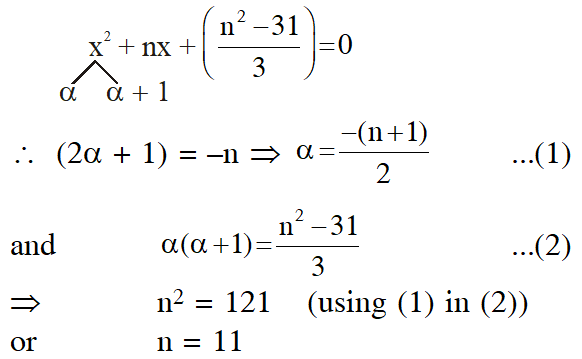Q. For any three positive real numbers a, b and $c, 9\left(25 a^{2}+b^{2}\right)+25\left(c^{2}-3 a c\right)=15 b(3 a+c)$ Then : (1) a, b and c are in G.P. (2) b, c and a are in G.P. (3) b, c and a are in A.P. (4) a, b and c are in A.P. [JEE(Main)-2017]
Ans. (3) $(15 a)^{2}+(3 b)^{2}+(5 c)^{2}-(15 a)(5 c)-(15 a)(3 b)-(3 b)(5 c)=0$ $\frac{1}{2}\left[(15 a-3 b)^{2}+(3 b-5 c)^{2}+(5 c-15 a)^{2}\right]=0$ it is possible when $15 a=3 b=5 c$ $\therefore b=\frac{5 c}{3}, a=\frac{c}{3}$ $a+b=2 c$ $\Rightarrow b, c, a$ in A.P.
Q. Let $a, b, c \in R .$ If $f(x)=a x^{2}+b x+c$ is such that $a+b+c=3$ and $f(x+y)=f(x)+f(y)+$ $\mathrm{xy}, \forall \mathrm{x}, \mathrm{y} \in \mathrm{R},$ then $\sum_{\mathrm{n}=1}^{10} \mathrm{f}(\mathrm{n})$ is equal to : (1) 255        (2) 330          (3) 165          (4) 190 [JEE(Main)-2017]
Ans. (2)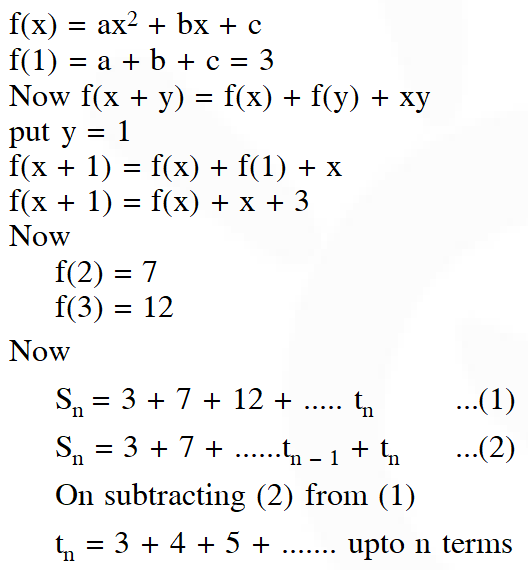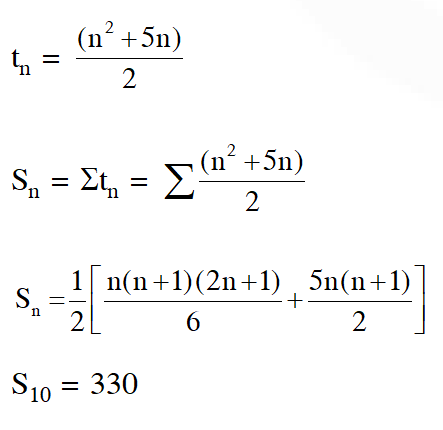Q. Let $a_{1}, a_{2}, a_{3}, \ldots ., a_{49}$ be in A.P. such that $\sum_{\mathrm{k}=0}^{12} \mathrm{a}_{4 \mathrm{k}+1}$ $=416$ and $a_{9}+a_{43}=66 .$ If $a_{1}^{2}+a_{2}^{2}+\ldots \ldots+a_{17}^{2}$ = 140m, then m is equal to- (1) 68 (2) 34 (3) 33 (4) 66 [JEE(Main)-2018]
Ans. (2)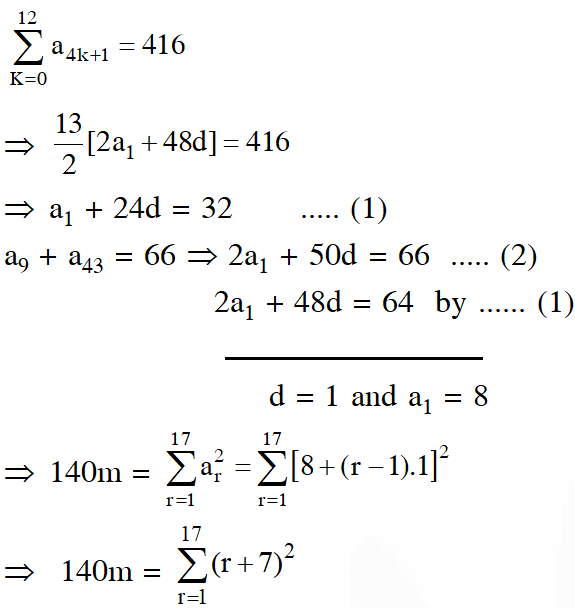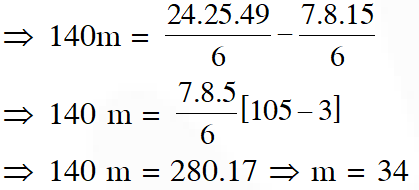Q. Let A be the sum of the first 20 terms and B be the sum of the first 40 terms of the series $1^{2}+2 \cdot 2^{2}+3^{2}+2 \cdot 4^{2}+5^{2}+2 \cdot 6^{2}+\ldots \ldots \ldots \quad$ If $\mathrm{B}-2 \mathrm{A}=100 \lambda,$ then $\lambda$ is equal to : (1) 248 (2) 464 (3) 496 (4) 232 [JEE(Main)-2018]
Ans. (1)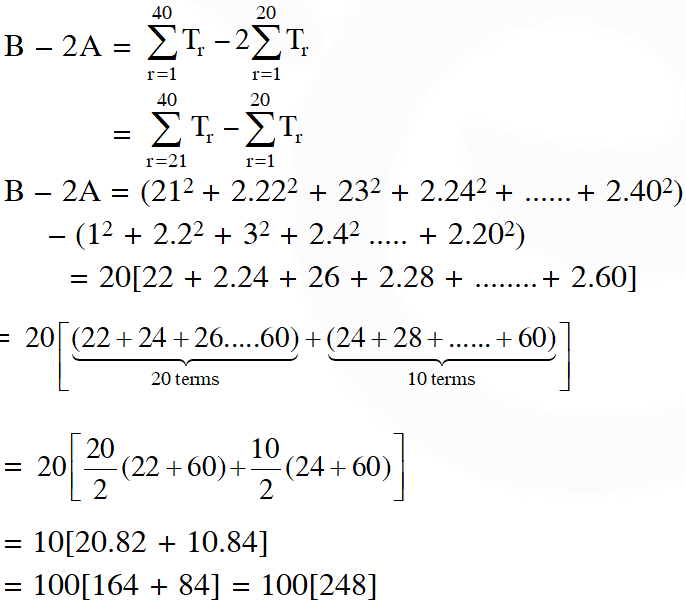Kritika
Aug. 22, 2023, 6:54 p.m.
Raja
March 11, 2023, 7:29 p.m.
In the last question,the solution is inaccurate.
Raja
March 11, 2023, 7:29 p.m.
In the last second
Aashna Khurana
July 23, 2022, 10:40 a.m.
Thank u E saral for providing these questions really thank u
Vijaydeep
March 16, 2022, 5:48 p.m.
Thank you 🙏
Kalmkaar raftaar
Jan. 29, 2022, 1:24 p.m.
Thnk. You eSaral
Mukkamala sumanth
Aug. 23, 2021, 3:36 p.m.
Thankuu broo
Heavy driver
June 6, 2021, 8:59 p.m.
Nice
Bhuvana
March 8, 2021, 7:04 p.m.
Update 2019,2020 questions also
sanobar
Feb. 17, 2021, 1:49 p.m.
but I am still trying . ................mission_______LETS KILL JEE %%%%%%%%
sanobar
Feb. 17, 2021, 1:45 p.m.
its too hard doode
swap
Dec. 16, 2020, 4:56 p.m.
cannot open solutions tho
swap
Dec. 16, 2020, 4:56 p.m.
nice
Amaan
Dec. 7, 2020, 12:02 a.m.
Subscription liya hai bhai eska?
Monu
Dec. 5, 2020, 12:30 a.m.
Nice helps a lot THANKS TO THE WHOLE eSARAL TEAM for their efforts
cristiano ronaldo
Nov. 26, 2020, 12:08 a.m.
where are 2020 and 2019 questions
Anonymous
Nov. 19, 2020, 5:02 p.m.
Now I can!
Anonymous
Nov. 19, 2020, 4:58 p.m.
Divyanshu
Oct. 19, 2020, 9:42 p.m.
Superb esaral ne jee or neet ke bachoo ke liye joo chapterwise privi previous year ke liye question ek jgh collect krke rkh rakhe h ye bht useful h superb esaral apne students ka kaam kr saral kr diya h
prashant chauhan
Sept. 29, 2020, 8:33 p.m.
nice
Bamniya Bro
Sept. 26, 2020, 12:16 p.m.
Excellent job
pallavi
Sept. 18, 2020, 7:28 p.m.
good
Monu
Sept. 16, 2020, 10:46 a.m.
Sept. 12, 2020, 7:12 p.m.
Gogious thanks
Manoj
Sept. 3, 2020, 8:36 a.m.
Ok
Goodboi
Sept. 1, 2020, 6:01 p.m.
Please Update this list and add 2019 and 2020 Questions also .
Anodit pandey
Aug. 27, 2020, 2:53 p.m.
It's helpful for me but these are very few que
divya
Aug. 20, 2020, 1:11 p.m.
nice
Pratyaksh
Aug. 14, 2020, 6:03 p.m.
Update it.
Abhishek kumawat
Aug. 6, 2020, 1:40 a.m.
Weldon
July 29, 2020, 3:22 p.m.
some questions and options are incorrect
Bhumika
July 26, 2020, 3:08 p.m.
Very nice
Dhanu
June 11, 2020, 3:27 p.m.
Super
Akhil
May 22, 2020, 9:02 a.m.
Chill bro
Anonymous
May 1, 2020, 10:07 a.m.
Thank you!